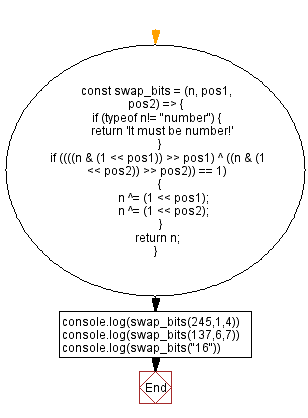# JavaScript - Swap two bits at given position in an integer

## JavaScript Bit Manipulation: Exercise-7 with Solution

Write a JavaScript program to swap two bits (from the right side, the rightmost position is 0) in the binary representation of an integer at the given position.

Test Data:
(245) -> 249

Explanation:
245 -> 11110101
Swap the 1st and 4th bits from the side of the said binary number.
11100111 -> 231
(137) -> 73
Explanation:
137 -> 10001001
Swap the 6th and 7th bits from the side of the said binary number.
01001001 -> 73

Sample Solution:

JavaScript Code:

``````const swap_bits = (n, pos1, pos2) => {
if (typeof n!= "number") {
return 'It must be number!'
}
if ((((n & (1 << pos1)) >> pos1) ^ ((n & (1 << pos2)) >> pos2)) == 1)
{
n ^= (1 << pos1);
n ^= (1 << pos2);
}
return n;
}
console.log(swap_bits(245,1,4))
console.log(swap_bits(137,6,7))
console.log(swap_bits("16"))
```
```

Sample Output:

```231
73
It must be number!
```

Flowchart:Live Demo:

See the Pen javascript-bit-manipulation-exercise-7 by w3resource (@w3resource) on CodePen.

* To run the code mouse over on Result panel and click on 'RERUN' button.*

Improve this sample solution and post your code through Disqus

What is the difficulty level of this exercise?

Test your Programming skills with w3resource's quiz.

﻿